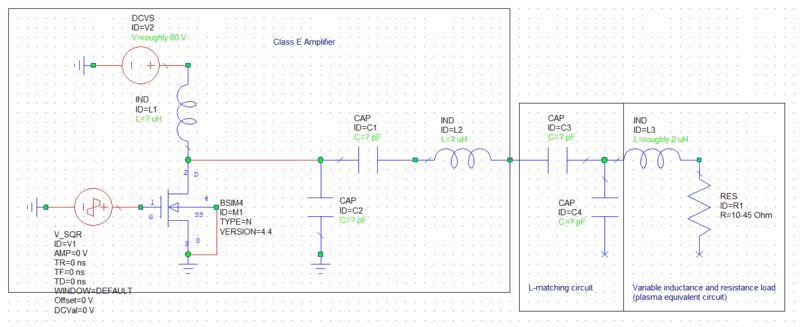• Mzzed

#### Mzzed

Hi all,

I am currently working on a project where the output/load consists of both a variable inductance and variable resistance, whilst the circuit driving this load is a class E radio frequency (RF) power amplifier with low resistance but relatively high/medium reactance (attempting to design an inductively coupled plasma source for those interested).

I have no problem matching the reactance of the variable inductor of the load with the reactance of the power amplifier using an impedance matching network with variable components. However I am unsure how to match the resistance (real part of the impedance) of the load with the resistance of the amplifier. The resistance of the amplifier is bellow 10 ohms whilst the load resistance can range anywhere from 10 to 45 ohms.

If i leave the system as is, the power transferred to the load will not be maximised, however if I somehow added some sort of variable resistance in the amplifier to match the load resistance, this would also be wasting power in the form of heat and would be pointless as i am trying to maximise power transfer to the load.

1) Is there a solution to my problem of being unable to match resistance of the amplifier and load? and if so how?

2) Will the larger load resistance cause the RF current/voltage to reflect back into the amplifier? or does that only occur due to reactive mismatch between amplifier and load?

Is your objective to match impedances just for the sake of matching? Or are you having a difficult time getting enough actual power into the load?

You can match the 10 to 45 Ohms of the load into the low resistance of the amplifier (say 4 Ohms) by using the L-match circuit.
First decide the resistance transformation ratio, for example 45/4 = 11. The take SQRT 11 = 3.3. This is the Q of your required matching network.
If the load had no reactance, you would then shunt the load with a reactance of 45/Q = 14 Ohms. You would place an opposite reactance of 14 Ohms ins series with the transmitter.
If the load is already reactive, you must first convert the R and X to parallel values using a Smith Chart or calculation. Then you design the matching network for this resistance.
You then find how much shunt reactance to add because it is in parallel with the Xp of the load.
Then you use an equal and opposite series reactance, but the value is in series with X of the amplifier, so must be adjusted accordingly.

•Mzzed
I'm not sure just what the objective is here. While it all good and well to have a matching network to cancel out reactance so the amplifier sees only a resistive load, there is no way to make the amplifier see a lower impedance without wasting more power in the amplifier. Maximum power transfer theorom states when maximum power transfer occurs but it does not say maximum efficiency occurs simultaneously.

•dlgoff and Mzzed
Thanks for the replies!

My goal is to transfer maximum power to the load. To do that I thought you had to match impedance which is a complex value that consists of both a real resistance and a reactance. I know you have to make the amplifier impedance equal to the conjugate of the load impedance, and I know how match the reactance using something like an L matching circuit. But I don't know how to match resistance to maximise power transfer since if I leave it as is, the resistances will not match and therefore the amplifier impedance will not be the conjugate of the load impedance, only the reactive components will cancel.

•Mzzed
Paging @berkeman
I was afraid you would do that...@Mzzed -- can you post the schematics of the drive amplifier and the load?

•dlgoff, Mzzed and Tom.G
Just quickly whipped this up, values are essentially undecided due to the above problem. Thanks for having a look for me.